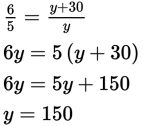# SAT Math Multiple Choice Question 833: Answer and Explanation

### Test Information

Question: 833

8. A sporting goods store ordered an equal number of white and yellow tennis balls. Thetennis ball company delivered 30 extra white balls, making the ratio of white ballsto yellow balls 6:5. How many tennis balls did the store originally order?

• A. 120
• B. 150
• C. 240
• D. 300

Explanation:

D

Difficulty: Medium

Category: Problem Solving and Data Analysis / Rates, Ratios, Proportions, and Percentages

Strategic Advice: When you're given a ratio and one amount of something, and asked to find another amount, setting up and solving a proportion usually gets you to the correct answer.

Getting to the Answer: Let the number of yellow balls received be y. Then the number of white balls received is 30 more than this, or y + 30. Set up a proportion (white to yellow) and cross-multiply to solve for y:Don't forget to check that you answered the right question (the number of balls ordered). Because the number of white balls ordered equals the number of yellow balls ordered, the total number of balls ordered is 2y, which is 2 × 150, or 300, making (D) correct.